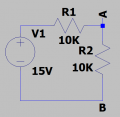# Thevenin of a voltage divider

#### MikeJacobs

Joined Dec 7, 2019
221
So i have not done this in a decade and trying to remember this. apparently im doing something wrong.

I was taught thevnins therom using the the short circuit method.

Vth = Isc*Rth

Take a simply voltage divider where R1 and R2 = 10k

The point of interest of A and B commonly called would be across R2.

So i was taught or at least i think i was to do the following.
Find ISC as if points A and B were shorted.

So in this case using a 15V supply i would have

15V / 10k = 1.5 mA is ISC

Then Vth would be what is the voltage at point A with A and B open, well, that in a voltage divider that is just the supply voltage of 15V.

So vth = 15V and Isc = 1.5 mA

So rth = 15V/1.5mA = 10k of course
So the eq circuit is a 15V supply with a 10 k resistor.

What did i do wrong here?

#### crutschow

Joined Mar 14, 2008
32,915
Sorry, I go lost between R2 and points A and B.
Schematics are the language of electronics.
Could you post a schematic of your circuit?
So the eq circuit is a 15V supply with a 10 k resistor.
For a voltage divider, the equivalent voltage would be the divider output voltage with no load, and its output resistance would be the value of the two resistors in parallel, so neither of those values appear to be correct.

Last edited:

#### MikeJacobs

Joined Dec 7, 2019
221
Typically people talk about a point of interest in the circuit where you want to know the equivalent. Sometimes called A and B

In this case, im defining points A and B across R2.
aka the normal place we talk about output on a voltage divider.

Make more sense?So as i was taught

Vth is the open circuit voltage from A to B
Isc is the current flowing when A and B is shorted

and Rth is
Vth / Isc = Rth

So where did i go wrong?

#### crutschow

Joined Mar 14, 2008
32,915
•click_here

#### MikeJacobs

Joined Dec 7, 2019
221
Which is 7.5V.
(Remember it's a voltage divider).
which is 1.5mA
Which is 7.5V / 1.5mA = 5kΩ.
Do you see now?
I dont get it
How is the open circuit voltage from A to B 7.5V?

Its open circuit, therefore no current flowing therefor no drop through R1?

#### crutschow

Joined Mar 14, 2008
32,915
How is the open circuit voltage from A to B 7.5V?
Open circuit means no load, not that R2 is disconnected.
So the voltage divider output with no load is 7.5V.

Last edited:

#### kaindub

Joined Oct 28, 2019
117
Both answers are correct. Its whether you consider R2 to be part of the analysis or not.

#### crutschow

Joined Mar 14, 2008
32,915
So is R2 part of the Thevenin circuit or an external load for the circuit.

#### kaindub

Joined Oct 28, 2019
117
You could consider it either way for this simple circuit. In more complex circuits you'd probably consider it as part of the source in order to calculate the following stages.